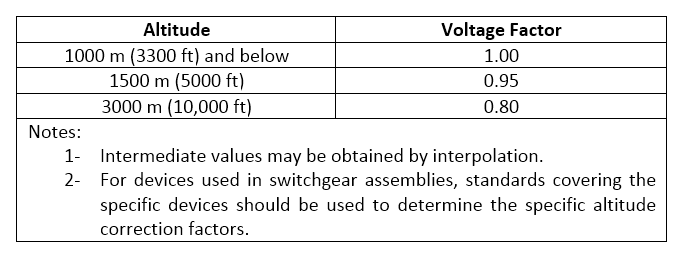﻿ Calculation of Altitude Correction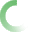The fields with the red border are required.# ALTITUDE CORRECTION FOR METAL-ENCLOSED CAPACITOR BANKS AND HARMONIC FILTER BANKS

The following calculator computes the altitude correction factor and resulting sea-level impulse withstand and power frequency withstand voltage requirements for electrical equipment being applied at altitudes greater than 1000 meters (3300 feet) in accordance with IEC and IEEE/ANSI standards.

ANSI/IEEE and IEC standards differ slightly on the standard available voltage ratings for components and equipment, impulse withstand voltage, and power frequency withstand voltage, as well as the methodology for de-rating these voltage ratings due to high altitude application. The following calculator provides both calculations based on the standards.

Be sure to use tables 1 and 2 to determine "industry standard" sea level withstand and impulse levels for IEC standards, and Table 3 for ANSI standards for your rated equipment voltage.

Calculator-1

Known variables: Equipment Elevation (meters or feet), Standard Equipment Impulse Voltage (kV), and Power Frequency Withstand Voltage (kV)

 Input Equipment Details* Input Equipment Elevation (in meters or feet): meters feet Input Sea Level Impulse Volage Based On System Voltage (kV): Input Sea Level Withstand Voltage Based on System Voltage (kV): * Obtain Impulse and Withstand Voltage Values from Tables 1 and 2 for IEC Standards, and Table 3 for ANSI Standards IEC Calculation Results IEC Correction Factor: Required Sea Level Impulse Voltage (kV): Required Sea Level Withstand Voltage (kV): ANSI/IEEE Calculation Results ANSI/IEEE Correction Factor: Required Sea Level Impulse Voltage (kV): Required Sea Level Withstand Voltage (kV):

Formulas and Variables

 IEC Correction Factor IEEE / ANSI Correction Factor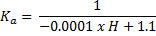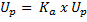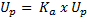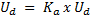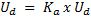Where:

Up = Rated lightning impule withstand voltage [kV (peak value)]

Ud = Rated short-duration power-frequency withstand voltage (kV (r.m.s. value))

Ur = Rated voltage (kV (r.m.s. value))

Ka = altitude correction factor

H = altitude above sea level in meters

Note: the correction factors should only be applied to the impulse and withstand voltages and not to the rated voltages.

Background

Medium-voltage metal-enclosed harmonic filter banks are designed and built with components that are based on application under "usual service conditions" as defined by IEC and ANSI standards. Generally, the "usual service conditions" defined in standards encompass ambient temperature, altitude, solar radiation, and environmental considerations.

The insulating property of air decreases as the altitude increases, therefore this phenomenon must be taken into account for air insulated components applied at altitudes greater than 1000 meters (3300 feet). ANSI/IEEE and IEC standards provide a methodology for determining the impulse withstand voltage, and power frequency withstand voltage for medium-voltage components used in metal-enclosed harmonic filter banks at high altitude.

IEC STANDARDS

In accordance with IEC 60694, the rated insulation level for switchgear and control gear is selected from the values given in tables 1 and 2, depending on location. In these tables, the withstand voltages are based on a standardized reference atmosphere (i.e. sea level). The standards state the rated withstand voltage values for lightning impulse voltage (Up) and power-frequency withstand voltage (Ud) shall be selected without crossing the horizontal marked lines based on the rated voltage of the switchgear or control gear, or in NEPSI's case, the harmonic filter or capacitor bank.

For most of the rated voltages, several rated insulation levels exists to allow for application of different overvoltage performance criteria. The choice of insulation level for a give rated voltage should be made considering the degree of exposure to fast-front and slow-front over-voltages, the type of neutral earthing of the system, and the type of overvoltage limiting devices. Medium-voltage metal-enclosed harmonic filter banks equipped with lightning arresters are considered to have a lower degree of exposure and therefore the lower withstand voltage is most often chosen. Metal-enclosed banks are considered to have lower exposer.

Table 1 - IEC Rated Insulation Levels for Rated Voltages
North American ApparatusTable 2 - IEC Rated Insulation Levels for Rated Voltages
For Non-North American ApparatusANSI / IEEE STANDARDS

ANSI standards are similar to IEC standards in that they both use a multiplying factor and both have standard voltage withstand ratings based on equipment voltage rating. Table 3 provides the standard voltage ratings for equipment rated in accordance with ANSI/IEEE standards. Table 3 can also be used in a similar manor to 1 and 2. The first column on the left is the Rated maximum Voltage (Ur). The second is the Power-frequency withstand voltage (Ud). The final column is the Impulse withstand (Up).

Table 4 is utilized to determine altitude correction factors in accordance altitude. The table shows a 1% decrease in withstand voltages for each 100 meters above 1000 meters. The voltage factors shown in Table 4 should be applied to both the power frequency withstand voltage and the impulse withstand voltage.

Table 3 - Standard Voltage and Insulation Levels for Components Rated in Accordance With ANSI/IEEE Standards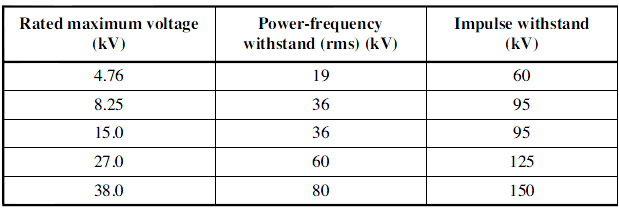Table 4 - Altitude Correction Factors